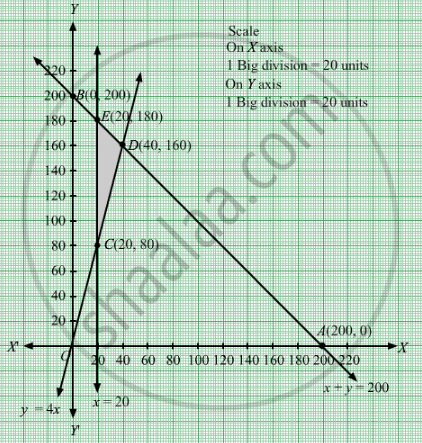# An Aeroplane Can Carry a Maximum of 200 Passengers. a Profit of Rs 400 is Made on Each First Class Ticket and a Profit of Rs 600 is Made on Each Economy Class Ticket. - Mathematics

Sum

An aeroplane can carry a maximum of 200 passengers. A profit of Rs 400 is made on each first class ticket and a profit of Rs 600 is made on each economy class ticket. The airline reserves at least 20 seats of first class. However, at least 4 times as many passengers prefer to travel by economy class to the first class. Determine how many each type of tickets must be sold in order to maximize the profit for the airline. What is the maximum profit.

#### Solution

Let  x tickets of first class and y tickets of economy class were sold.
Number of tickets cannot be negative.
Therefore, $x, y \geq 0$

An aeroplane can carry a maximum of 200 passengers.

$x + y \leq 200$
The airline reserves at least 20 seats of first class and at least 4 times as many passengers prefer to travel by economy class to the first class.
$x \geq 20$
$y \geq 4x$
A profit of Rs 400 is made on each first class
ticket and a profit of Rs 600 is made on each economy class ticket. Therefore, profit gained by selling x tickets of first class and tickets of economy class is Rs 400x and Rs 600y respectively.
Total profit = Z = $400x + 600y$ which is to be maximised
Thus, the mathematical formulat​ion of the given linear programmimg problem is
Max Z =  $400x + 600y$
subject to $x + y \leq 200$
$x \geq 20$
$y \geq 4x$
$x, y \geq 0$
First we will convert inequations into equations as follows:
+ y =200, x = 20, = 4xx = 0 and y = 0

Region represented by x y ≤ 200:
The line x + y = 200 meets the coordinate axes at A(200, 0) and B(0, 200) respectively.
By joining these points we obtain the line
x + y = 200. Clearly (0,0) satisfies the inequation x y ≤ 200. So,the region which contains the origin represents the solution set of the inequation x y ≤ 200.

Region represented by x  ≥ 20:
The line x  = 20 is the line that passes through (20, 0) and is parallel to Y axis.The region to the right of the line x  = 20 will satisfy the inequation x  ≥ 20.
Region represented by  ≥ 4x:
The line y = 4x is the line that passes through (0, 0).The region above the line y = 4x will satisfy the inequation ≥ 4x.Let us understand by taking one point which is below the line y = 4x. Let it be (1, 3).Here, 3 < 4 which does not satisfy the inequation  ≥ 4x. Hence, the region above the line y = 4x will satisfy the inequation  ≥ 4x.

Region represented by ≥ 0 and y ≥ 0:
Since, every point in the first quadrant satisfies these inequations. So, the first quadrant is the region represented by the inequations x ≥ 0, and y ≥ 0.

The feasible region determined by the system of constraints x y ≤ 200, x  ≥ 20, y  ≥ 4x,x ≥ 0 and y ≥ 0 are as follows.The corner points are C(20, 80), D(40, 160), E(20, 180).
The values of Z at these corner points are as follows

 Corner point Z = 400x + 600y C 56000 D 112000 E 116000

The maximum value of Z is 116000 which is attained at C(20, 180).
Thus, the maximum profit is Rs 116000 obtained when 20 first class tickets and 180 economy class tickets were sold
Concept: Graphical Method of Solving Linear Programming Problems
Is there an error in this question or solution?

#### APPEARS IN

RD Sharma Class 12 Maths
Chapter 30 Linear programming
Exercise 30.4 | Q 22 | Page 53